Go to: CoHort Software | CoPlot

# CoPlot's Graph Objects (plot data and equations on XY, 3D, triangle, and polar graphs, and on orthographic, mercator, and conic maps)

### Great Scientific Graphs

CoPlot's graphs have many features which were designed specifically to meet the needs of scientists and engineers, includingThese are described and illustrated below. Since graphs are one of the standard types of drawing objects in CoPlot, you can put as many Graph objects on a drawing as you want.

Go to: CoHort Software | CoPlot | Top

### 7 Graph Types

CoPlot has 7 basic graph types: XY, 3D, Triangle, Polar, Orthographic, Mercator, and Conic. On each graph, you can plot as many datasets and equations as you want. A legend is generated automatically for the graph, but you can control its location and appearance.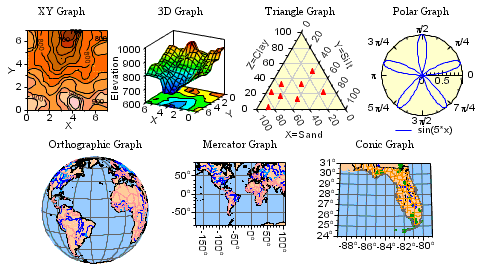• XY Graph - XY is the most common type of graph.
• 3D Graph - The 3D graph can be rotated to any angle and tilted up or down so that you can get any view of the graph.
• Triangle Graphs - are useful for plotting various mixtures with 3 components (for example, soil is made up of sand, silt, and clay). There is an option in the program to change the axis arrangement of triangle graphs (for example, for USDA soil classification charts).
• Polar Graphs - plot data and equations in polar coordinates (angle and radius, instead of x and y).
• Orthographic - is a common map projection for the entire world.
• Mercator - is a common map projection for the entire world or portions of it.
• Conic - is a common map projection for smaller portions of the world.

Maps - The Orthographic, Mercator, and Conic graph types are common map projections. CoPlot comes with special world map data files so you can generate maps of all or part of the world with these graph types. CoPlot also comes with special detailed map data files for the U.S. (derived from the USGS Digital Line Graph Data) with lake, river, state, county, city, park, and road information (but not city street information), so you can make maps of areas as small as a few miles across.

But otherwise, the Orthographic, Mercator, and Conic graph types are just like other types of graphs, so you can plot your latitude longitude data on top of the map data. Since you can set the axis ranges to any values you want, you can easily make a map of any part of the world. There is even a "wizard" (Graph : Make Map) that lets you specify a place name (for example, "Germany") and then makes a map centered on that place.

Go to: CoHort Software | CoPlot | Top

### 40 Data Representations

For each dataset on each graph, you will specify an X column, a Y column, perhaps a Z column, and a set of attributes describing how the data should be plotted. There are about 40 basic data 'Representations' (ways to draw the data, including lines, markers, filled areas, various types of bars, contour lines, 3D surfaces, etc.). The Representations can be further customized in an infinite number of ways by changing the color, line type, line width, marker type, fill type, background color, etc.).

Here are some of the line and marker representations. These graphs also show some variations of the graph axis attributes.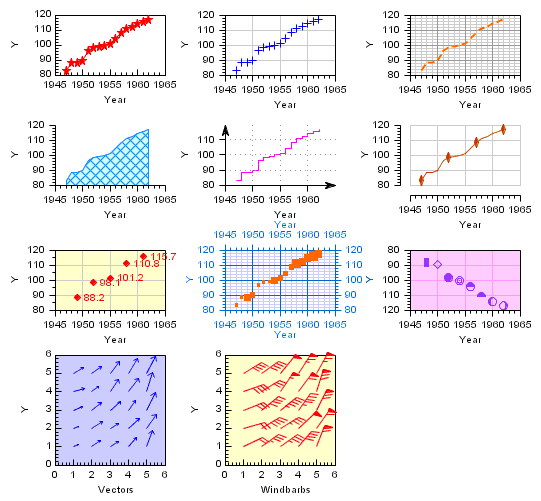Here are some of the surface representations. ("Contour Lines" is somewhat cluttered because the graph is so small.)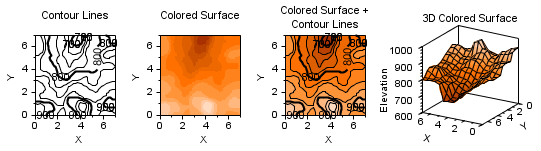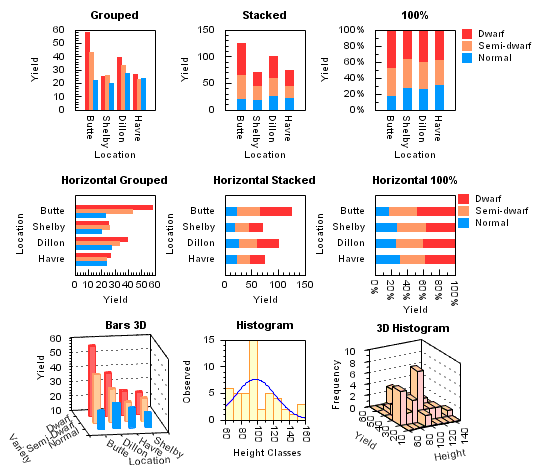Go to: CoHort Software | CoPlot | Top

### 18 Equation Representations

For each equation that you plot on each graph, you will specify the actual equation and a set of attributes describing how the equation should be plotted. On any type of graph, the equation can reference x (for example, "0.1 + 1.3*x - 0.9*x^2"). On contour and 3D graphs, the equation can reference x and y (for example, "0.1 + 1.3*x - 0.2*y + 0.9*x*y"). Equations can use the many built-in functions (for example, abs, acos, asin, atan, cos, cumNorm, ln, log, norm, round, sign0, sign1, sin, sqr, sqrt, tan, trunc) and constants (e, pi). There are 18 equation 'Representations' (ways to draw the equation, including: lines, fill above, fill below, fill to zero, variations of contour lines, and variations of 2D and 3D surfaces). The Representations can be further customized in an infinite number of ways by changing the color, line type, line width, fill type, background color, etc.).

Here are some of the equation representations.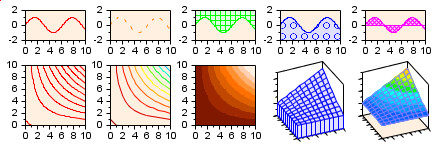Go to: CoHort Software | CoPlot | Top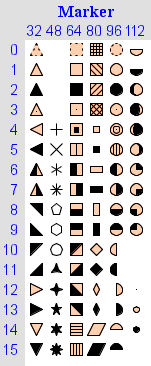### Flexible Attributes

Each dataset, each equation, and each other part of the graph (for example, the X Axis primary tick marks, or the Y Axis grid lines) can be further customized by changing their color, line type, line width, marker type, fill type, background color, etc. For example, the line width for one dataset might be different from the line width for another dataset.
• Color - You can pick colors from a pre-defined palette of 142 colors, or you can specify any of 16.7 million custom colors (256 levels of red, green, and blue).
• Line Width - You can set the width of any line to any width (not just a few preset line widths).
• Line Types - You can select the dot-dash pattern from over 30 different dot-dash patterns. And you can even specify how long the dots and dashes are.
• Marker Types - For each dataset, you can choose from 90 different marker types (see samples to the right). Or, you can use any character in any font (for example, ASCII, Greek, Map symbols, and Weather symbols).

Go to: CoHort Software | CoPlot | Top

### Asymmetric and Horizontal Error Bars

The upper and lower error values are specified separately, so you can have asymmetric error bars or turn off individual error bars. You can also specify right and left error values and get horizontal error bars. And you can turn off the caps at the ends of the error bars.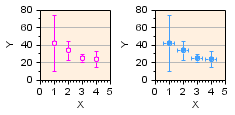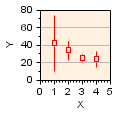Go to: CoHort Software | CoPlot | Top

### 12 Axis Types

For each axis on each graph, you can choose from 12 different types of axes: linear, log, pi, date, time, degrees, normal probability, standard deviation, probit, etc. For example, this makes it easy to make a log-log graph.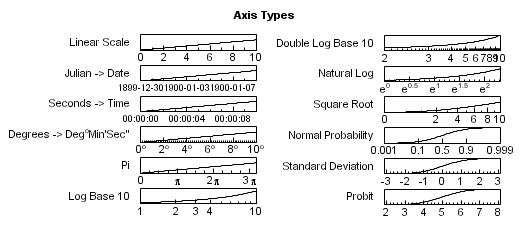Go to: CoHort Software | CoPlot | Top

### 150 Number Formats

For axis labels and marker labels, you can choose how numbers are formatted. There are 19 format categories (general format, scientific notation, engineering notation, date, time, degree, multiple of pi, hexadecimal, binary, etc.) and over 150 total formats. Many people outside the U.S. will be happy to hear that you can choose whether to use a period or a comma as the decimal point.

Go to: CoHort Software | CoPlot | Top